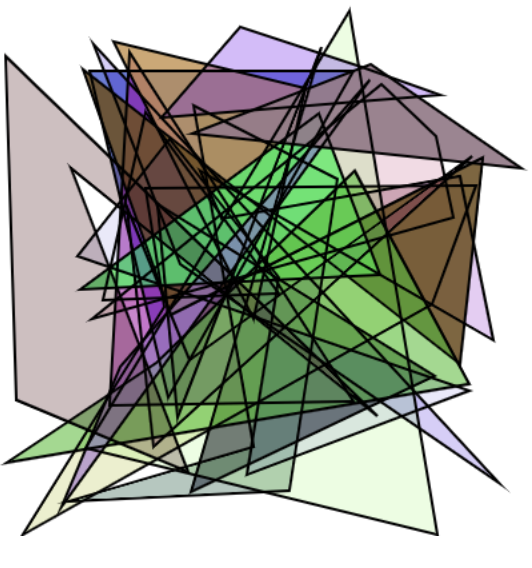# Drawing simple shapes#

Note

Note that because we are exposing the Web Canvas API, you can find more tutorials and documentation following this link: https://developer.mozilla.org/en-US/docs/Web/API/Canvas_API

There are some API differences though:

• The Canvas widget is directly exposing the CanvasRenderingContext2D API

• All the API is written in snake_case instead of camelCase, so for example `canvas.fillStyle = 'red'` in JavaScript becomes `canvas.fill_style = 'red'` in Python

Before we can start drawing, we need to talk about the canvas grid. The origin of this grid is positioned in the top left corner at coordinate (0,0). All elements are placed relative to this origin. So the position of the top left corner of the blue square becomes x pixels from the left and y pixels from the top, at coordinate (x,y).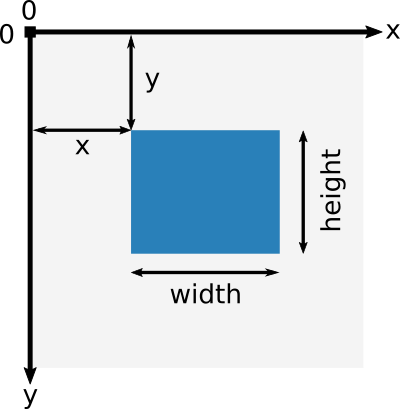## Drawing rectangles#

There are six methods that draw rectangles on the canvas:

• `fill_rect(x, y, width, height=None)`:

Draws a filled rectangle. If `height` is None, it is set to the same value as `width`.

• `stroke_rect(x, y, width, height=None)`:

Draws a rectangular outline. If `height` is None, it is set to the same value as `width`.

• `fill_rects(x, y, width, height=None)`:

Draws filled rectangles. Where `x`, `y`, `width` and `height` are either integers, lists of integers or NumPy arrays. If `height` is None, it is set to the same value as `width`.

• `stroke_rects(x, y, width, height=None)`:

Draws rectangular outlines. Where `x`, `y`, `width` and `height` are either integers, lists of integers or NumPy arrays. If `height` is None, it is set to the same value as `width`.

• `fill_styled_rects(x, y, width, height, color, alpha)`:

Same as `fill_rects` but with additional `(n x 3)` `color` ndarray and `(n)` `alpha` ndarray.

• `stroke_styled_rects(x, y, width, height, color, alpha)`:

Same as `stroke_rects` but with additional `(n x 3)` `color` ndarray and `(n)` `alpha` ndarray.

You can also clear a certain canvas rectangle area:

• `clear_rect(x, y, width, height=None)`:

Clears the specified rectangular area, making it fully transparent. If `height` is None, it is set to the same value as `width`.

```from ipycanvas import Canvas

canvas = Canvas(width=200, height=200)

canvas.fill_rect(25, 25, 100, 100)
canvas.clear_rect(45, 45, 60, 60)
canvas.stroke_rect(50, 50, 50, 50)

canvas
````fill_rects` and `stroke_rects` are blazingly fast ways of drawing up to a million rectangles at once:

```import numpy as np

from ipycanvas import Canvas

n_particles = 100_000

x = np.array(np.random.rayleigh(250, n_particles), dtype=np.int32)
y = np.array(np.random.rayleigh(250, n_particles), dtype=np.int32)
size = np.random.randint(1, 3, n_particles)

canvas = Canvas(width=800, height=500)

canvas.fill_style = "green"
canvas.fill_rects(x, y, size)

canvas
```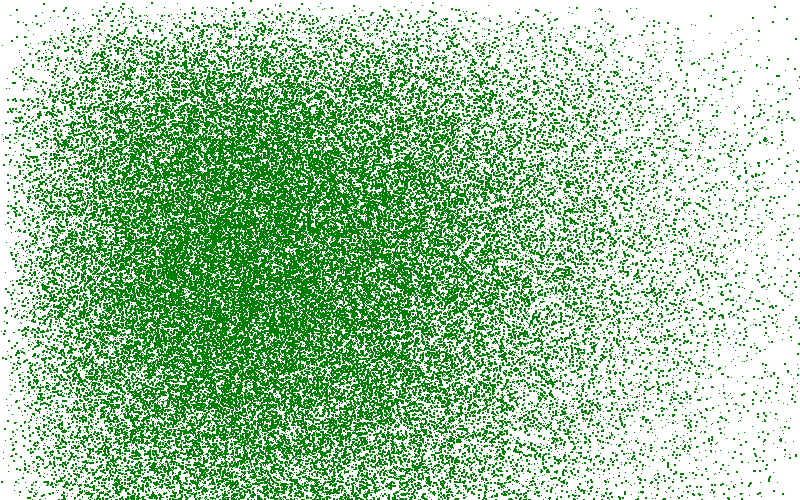## Drawing polygons#

You can draw a polygon by providing a list of points, either a Python list, or a NumPy array. It’s the fastest way to draw a polygon with ipycanvas.

• `fill_polygon(points)`:

Fill a polygon from a list of points `[(x1, y1), (x2, y2), ..., (xn, yn)]`.

• `stroke_polygon(points)`: Draw polygon outline from a list of points `[(x1, y1), (x2, y2), ..., (xn, yn)]`.

• `fill_polygons(points, points_per_polygon=None)`:

Fill multiple polygons at once.

• `stroke_polygons(points, points_per_polygon=None)`:

Stroke multiple polygons at once. See Polygons / line-segments for details.

• `fill_styled_polygons(points, color, alpha, points_per_polygon=None)`:

Fill multiple polygons at once where each polygon can have its own color. See Polygons / line-segments for details.

• `stroke_styled_polygons(points, color, alpha, points_per_polygon=None)`:

Stroke multiple polygons at once where each polygon can have its own color. See Polygons / line-segments for details.

```from ipycanvas import Canvas

canvas = Canvas(width=200, height=200)

canvas.fill_style = "#63934e"
canvas.stroke_style = "#4e6393"
canvas.line_width = 5
canvas.fill_polygon([(20, 20), (180, 20), (100, 150)])
canvas.stroke_polygon([(20, 20), (180, 20), (100, 150)])

canvas
```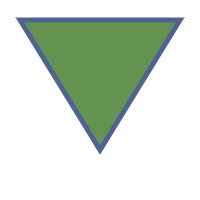```from math import pi
import numpy as np
from ipycanvas import Canvas

def polygon(canvas, x, y, radius, n_points):
angles = (2 * pi / n_points) * np.arange(n_points)

v_x = x + np.cos(angles) * radius
v_y = y + np.sin(angles) * radius

points = np.stack((v_x, v_y), axis=1)

canvas.fill_polygon(points)

background_color = "#89c64f"
polygon_color = "#c6574f"

canvas = Canvas(width=200, height=200)

canvas.fill_style = background_color
canvas.fill_rect(0, 0, canvas.width, canvas.height)

canvas.fill_style = polygon_color
polygon(canvas, 100, 100, 70, 6)

canvas
```## Drawing arcs and circles#

There are methods that draw arcs/circles on the canvas:

• `fill_arc(x, y, radius, start_angle, end_angle, anticlockwise=False)`:

Draw a filled arc centered at `(x, y)` with a radius of `radius`.

• `stroke_arc(x, y, radius, start_angle, end_angle, anticlockwise=False)`:

Draw an arc outline centered at `(x, y)` with a radius of `radius`.

• `fill_arcs(x, y, radius, start_angle, end_angle, anticlockwise=False)`:

Draw filled arcs centered at `(x, y)` with a radius of `radius`. Where `x`, `y`, `radius` and other arguments are NumPy arrays, lists or scalar values.

• `stroke_arcs(x, y, radius, start_angle, end_angle, anticlockwise=False)`:

Draw an arc outlines centered at `(x, y)` with a radius of `radius`. Where `x`, `y`, `radius` and other arguments are NumPy arrays, lists or scalar values.

• `fill_styled_arcs( x, y, radius, start_angle, end_angle, color, alpha, anticlockwise=False)`:

Same as `fill_arcs` but with additional `(n x 3)` `color` ndarray and `(n)` `alpha` ndarray.

• `stroke_styled_arcs( x, y, radius, start_angle, end_angle, color, alpha, anticlockwise=False)`:

Same as `stroke_arcs` but with additional `(n x 3)` `color` ndarray and `(n)` `alpha` ndarray.

• `fill_circle(x, y, radius)`:

Draw a filled circle centered at `(x, y)` with a radius of `radius`.

• `stroke_circle(x, y, radius)`:

Draw an circle outline centered at `(x, y)` with a radius of `radius`.

• `fill_circles(x, y, radius)`:

Draw filled circles centered at `(x, y)` with a radius of `radius`. Where `x`, `y`, `radius` are NumPy arrays, lists or scalar values.

• `stroke_circles(x, y, radius)`:

Draw a circle outlines centered at `(x, y)` with a radius of `radius`. Where `x`, `y`, `radius` are NumPy arrays, lists or scalar values.

• `fill_styled_circles( x, y, radius color, alpha)` :

Same as `fill_circles` but with additional `(n x 3)` `color` ndarray and `(n)` `alpha` ndarray.

• `stroke_styled_circles( x, y, radius, color, alpha)` :

Same as `stroke_circles` but with additional `(n x 3)` `color` ndarray and `(n)` `alpha` ndarray.

```from math import pi

from ipycanvas import Canvas

canvas = Canvas(width=200, height=200)

canvas.fill_style = "red"
canvas.stroke_style = "blue"

canvas.fill_arc(60, 60, 50, 0, pi)
canvas.stroke_circle(60, 60, 40)

canvas
```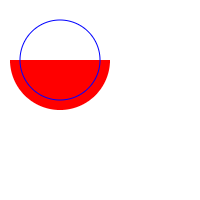## Drawing lines#

There are two commands for drawing a straight line from one point to another:

• `stroke_line(x1, y1, x2, y2)`:

Draw a line from `(x1, y1)` to `(x2, y2)`.

• `stroke_lines(points)`:

Draw a path of consecutive lines from a list of points `[(x1, y1), (x2, y2), ..., (xn, yn)]`.

• `stroke_styled_line_segments(points, points_per_line_segment=None)`:

Draw multiple disconnected line-segments at once. See Polygons / line-segments for details.

• `stroke_styled_line_segments(points, color, alpha, points_per_line_segment=None)`:

Draw multiple disconnected line-segments at once. See Polygons / line-segments for details.

```from ipycanvas import Canvas

canvas = Canvas(width=200, height=200)

canvas.stroke_style = "blue"
canvas.stroke_line(0, 0, 150, 150)

canvas.stroke_style = "red"
canvas.stroke_line(200, 0, 0, 200)

canvas.stroke_style = "green"
canvas.stroke_line(150, 150, 0, 200)

canvas
```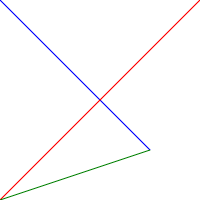```import numpy as np

from ipycanvas import Canvas

canvas = Canvas(width=200, height=200)

n = 50
x = np.linspace(0, 200, n)
y = np.random.randint(200, size=n)

points = np.stack((x, y), axis=1)

canvas.stroke_lines(points)

canvas
```## Vectorized methods#

Most methods like `fill_rect`/`stroke_rect` and `fill_circle`/`stroke_circle` have vectorized counterparts: `fill_rects`/`stroke_rects` and `fill_circles`/`stroke_circles`. It is essential to use those methods when you want to draw the same shape multiple times with the same style.

For example, it is way faster to run:

```from ipycanvas import Canvas

canvas = Canvas(width=300, height=300)

canvas.global_alpha = 0.01

size = [i for i in range(300)]
position = [300 - i for i in range(300)]

canvas.fill_rects(position, position, size)

canvas
```

```from ipycanvas import Canvas

canvas = Canvas(width=300, height=300)

canvas.global_alpha = 0.01

for i in range(300):
size = i
position = 300 - i

canvas.fill_rect(position, position, size)

canvas
```

## Styled vectorized methods#

Ipycanvas provides methods to draw the same shape multiple times but with different colors:

• `fill_styled_rects` / `stroke_styled_rects`

• `fill_styled_circles` / `stroke_styled_circles`

• `fill_styled_arcs` / `stroke_styled_arcs`

• `fill_styled_polygons` / `stroke_styled_polygons`

• `fill_styled_line_segments` / `stroke_styled_line_segments`

### Rects#

```import numpy as np
from ipycanvas import Canvas, hold_canvas

canvas = Canvas(width=400, height=300)
n_rects = 300
x = np.random.randint(0, canvas.width, size=(n_rects))
y = np.random.randint(0, canvas.width, size=(n_rects))
width = np.random.randint(10, 40, size=(n_rects))
height = np.random.randint(10, 40, size=(n_rects))
colors_fill = np.random.randint(0, 255, size=(n_rects, 3))
colors_outline = np.random.randint(0, 255, size=(n_rects, 3))
alphas = np.random.random(n_rects)
with hold_canvas():
canvas.fill_styled_rects(x, y, width, height, color=colors_fill, alpha=alphas)
canvas.line_width = 2
canvas.stroke_styled_rects(x, y, width, height, color=colors_outline, alpha=alphas)
canvas
```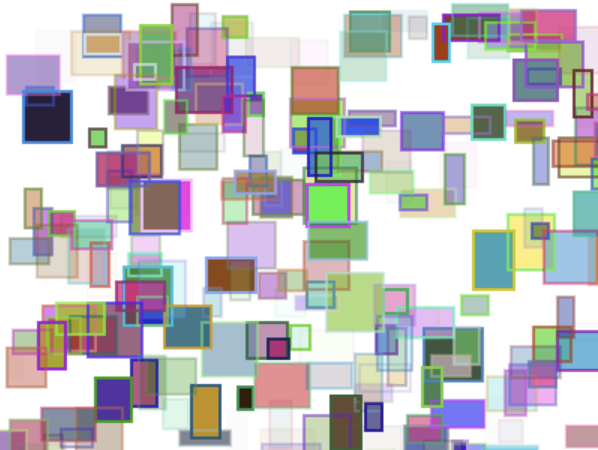### Circles#

```import numpy as np
from ipycanvas import Canvas, hold_canvas

canvas = Canvas(width=300, height=300)
n_circles = 100
x = np.random.randint(0, canvas.width, size=(n_circles))
y = np.random.randint(0, canvas.width, size=(n_circles))
r = np.random.randint(10, 20, size=(n_circles))
colors_fill = np.random.randint(0, 255, size=(n_circles, 3))
colors_outline = np.random.randint(0, 255, size=(n_circles, 3))
alphas = np.random.random(n_circles)
with hold_canvas():
canvas.fill_styled_circles(x, y, r, color=colors_fill, alpha=alphas)
canvas.line_width = 2
canvas.stroke_styled_circles(x, y, r, color=colors_outline)
canvas
```### Arcs#

```import numpy as np
from ipycanvas import Canvas, hold_canvas
import math

canvas = Canvas(width=300, height=300)
n_circles = 100
x = np.random.randint(0, canvas.width, size=(n_circles))
y = np.random.randint(0, canvas.width, size=(n_circles))
r = np.random.randint(10, 20, size=(n_circles))
start_angle = np.random.randint(0, 360, size=(n_circles))
end_angle = np.random.randint(0, 360, size=(n_circles))
start_angle = 0
end_angle = math.pi
start_angle = np.random.random(n_circles) * math.pi
end_angle = np.random.random(n_circles) * math.pi
alphas = np.random.random(n_circles)
with hold_canvas():
canvas.fill_style = "cyan"
canvas.fill_arcs(x, y, r, start_angle, end_angle)
canvas.line_width = 1
canvas.stroke_style = "black"
canvas.stroke_arcs(x, y, r, start_angle, end_angle)
canvas
```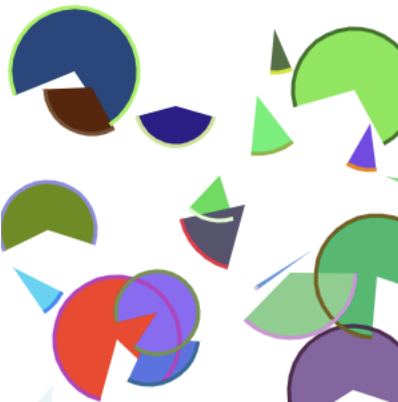### Polygons / line-segments#

#### Case 1: All polygons / line-segments have the same number of points#

```import numpy as np
from ipycanvas import Canvas, hold_canvas

canvas = Canvas(width=300, height=300)
n_polygons = 50

# each polygon has 4 points
n_points_per_polygon = 4

polygons = np.zeros([n_polygons, n_points_per_polygon, 2])

polygons[:, 0, 0] = 0.0
polygons[:, 0, 1] = 0.0

polygons[:, 1, 0] = 1.0
polygons[:, 1, 1] = 0.0

polygons[:, 2, 0] = 1.0
polygons[:, 2, 1] = 1.0

polygons[:, 3, 0] = 0.0
polygons[:, 3, 1] = 1.0

colors_fill = np.random.randint(0, 255, size=(n_polygons, 3))
colors_outline = np.random.randint(0, 255, size=(n_polygons, 3))

# scale each polygon
polygons *= np.linspace(1.0, 200.0, num=n_polygons)[:, None, None]

# translate each polygon
polygons += np.linspace(1.0, 100.0, num=n_polygons)[:, None, None]

points_per_polygon = np.ones([n_polygons]) * n_points_per_polygon
with hold_canvas():
canvas.stroke_styled_polygons(polygons, color=colors_fill)
canvas
```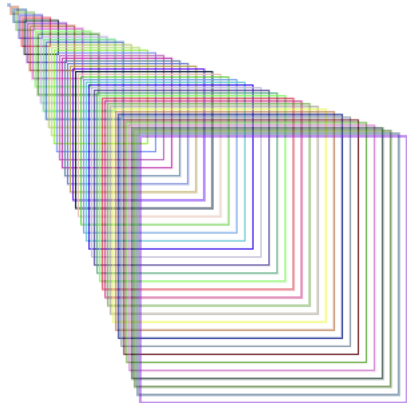```import numpy as np
from ipycanvas import Canvas, hold_canvas

canvas = Canvas(width=300, height=300)

n_line_segments = 20

n_points_per_line_segment = 500

line_segments = np.zeros([n_line_segments, n_points_per_line_segment, 2])

x = np.linspace(0, canvas.width, num=n_points_per_line_segment)[None, :]
line_segments[:, :, 0] = np.linspace(0, canvas.width, num=n_points_per_line_segment)[
None, :
]
line_segments[:, :, 1] = (30.0 * np.sin(x * 0.1))[None, :]

colors_outline = np.random.randint(0, 255, size=(n_polygons, 3))

# translate line segments in y direction
line_segments[:, :, 1] += np.linspace(1.0, canvas.height, num=n_line_segments)[:, None]

with hold_canvas():
canvas.stroke_styled_line_segments(line_segments, color=colors_fill)
canvas
```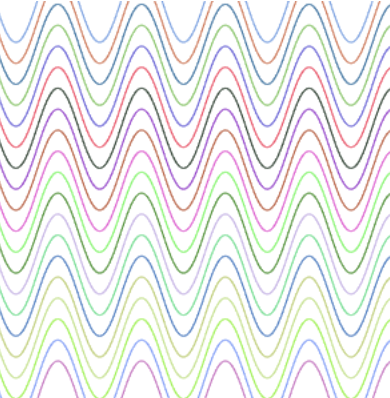#### Case 2: Polygons / line-segments can have different number of Points.#

Polygons can be given as a list of ndarrays:

```import numpy as np
from ipycanvas import Canvas, hold_canvas

canvas = Canvas(width=400, height=400)

triangle = [(0, 0), (0, 40), (30, 40)]  # triangle
rectangle = [(100, 100), (300, 100), (300, 200), (100, 200)]  # rectangle
irregular = np.random.randint(0, 400, size=(5, 2))  # irregular with 5 sides
polygons = [triangle, rectangle, irregular]
colors = [(255, 0, 0), (0, 255, 0), (0, 0, 255)]

with hold_canvas():
canvas.fill_styled_polygons(polygons, color=colors)
canvas
```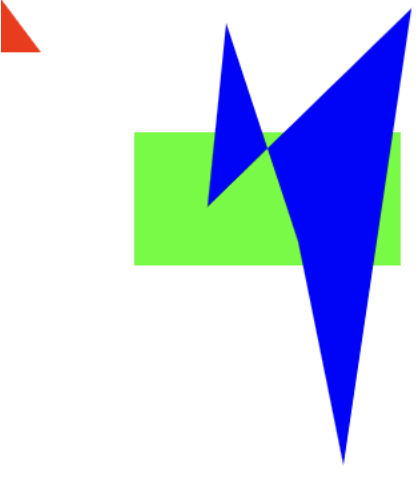Polygons can be given as a flat ndarray:

```import numpy as np
from ipycanvas import Canvas, hold_canvas

canvas = Canvas(width=400, height=400)
n_polygons = 20
points_per_polygon = np.random.randint(3, 6, size=n_polygons)
total_points = np.sum(points_per_polygon)
polygons = np.random.randint(0, 400, size=[total_points, 2])
alpha = np.random.random(n_polygons)
colors_fill = np.random.randint(0, 255, size=(n_polygons, 3))
colors_outline = np.random.randint(0, 255, size=(n_polygons, 3))

with hold_canvas():
# the filling
canvas.fill_styled_polygons(
polygons, points_per_polygon=points_per_polygon, color=colors_fill, alpha=alpha
)

# draw outlines ontop where each line has the same style
canvas.stroke_style = "black"
canvas.line_width = 2
canvas.stroke_polygons(polygons, points_per_polygon=points_per_polygon)
canvas
```